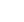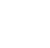# Introductory Statistics : Sample Survey and Instruments for Statistical Inference

## Description

In this course, you will learn about sample surveys with the concepts of samples and populations. In addition, we will discuss possible problems(bias) of the surveys based on practical examples and concept of probability errors in sampling. This course will familiarize you with statistical inference, the process by which data is used to draw a conclusion or uncover a scientific truth about a population from a sample. You can learn how to obtain confidence intervals and estimate the population percentage using sample percentage. We will also cover statistical inferences using sample average to estimate the population average with the concept of standard error and measurement error. Finally, you will learn fundamental idea and process of significance test to determine whether the observed difference is real or simply accidental. Test for difference in average between two groups and Chi-Square test will be discussed in depth in this course.

## Specific details

Category of Education Physical Science

## University

Seoul National University

There are no comments posted here yetinfo@educom.net
 About Terms and ConditionsPrivacy PolicyRankingsAccreditations